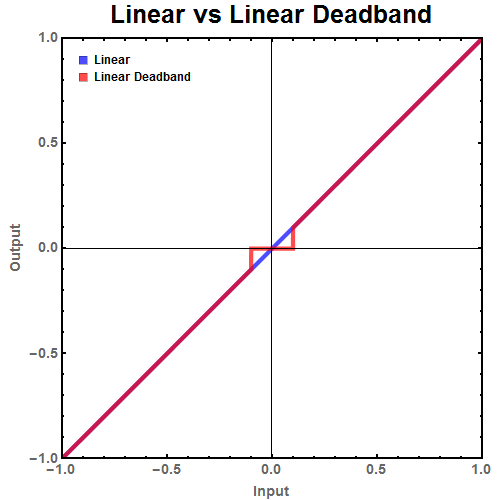In our robot code, we make use of deadbands to account for the fact that our joysticks drift slightly.

In previous years, we just did a simple cutoff of our values:

``````// Constants

// Loop
double rotation = oi.getTurn();
rotation = (Math.abs(rotation) > deadband)? 0.0 : rotation;
``````

Although this worked, it raised the following problem that was mentioned by mimirgames. This causes a hard cut in the motor input. For example, with a deadband of `0.1`: when the joystick reads `0.09` the output will be `0.0%`, but with a reading of `0.11`, the output will be `0.11%`. While this does not seem too bad, it causes a loss of fine control from the driver’s input. They can only go `0.0%` or `0.1%`. Nothing in between.## The Solution

For most inputs, small changes of small values matter much more than small changes of big values. For example, going 5 mph faster then intended is much more serious when parking a car than when one is driving on the freeway (source: mimirgames).

To achive this, we use this “fancy equation”. Essentially, we pass our input through a cubic scaling funciton:

``````w = precision //(0 <= w <= 1)
d = deadband //(0 <= d <= 0.9)
x = joystick_input //(-1 <= x <= 1)

output = ((w * (x ^ 3)  + (1.0 - w) * x) - (abs(x) / x) * (w * (d ^ 3) + (1.0 - w) * d)) / (1.0 - (w * (d ^ 3) + (1.0 - w) * d))
``````

## Visualization

This visualization of our cubic scaling function can be interacted with via the two labled sliders. To view the full Desmos project, click here